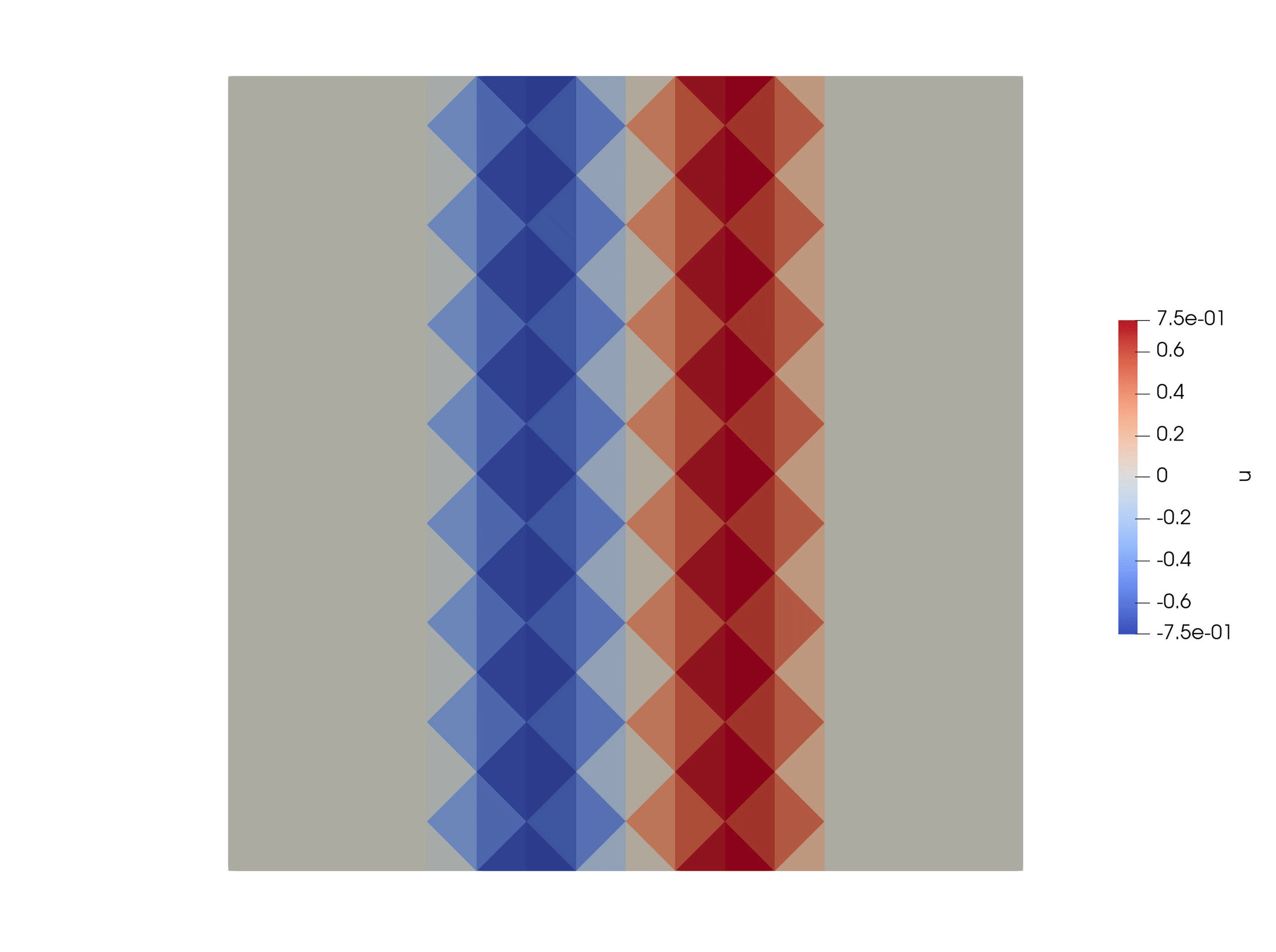# Initial Conditions

Currently we provide the following initial conditions:

## Zero

All quantities are set to zero. This is the standard case to work with point sources or dynamic rupture.

## Planar wave

A planar wave for convergence tests. The inital values are computed such that a planar wave in a unit cube is imposed. For elastic, anisotropic and viscoelastic materials, we impose a P and an S wave travelling in opposite directions. For poroelastic materials, we impose a slow P and an S wave travelling in one direction and a fast P wave travelling in opposite direction. This scenario needs periodic boundary conditions to make sense. This is the only case where the old netcdf mesh format is prefered. After the simulation is finished the errors between the analytic solution and the numerical one are plotted in the $$L^1$$-, $$L^2$$- and $$L^\infty$$-norm.

Use cube_c to generate the meshes for the convergence tests: https://github.com/SeisSol/SeisSol/tree/master/preprocessing/meshing/cube_c

## Superimposed planar wave

Superimposed three planar waves travelling in different directions. This is especially interesting in the case of directional dependent properties such as for anisotropic materials.

## Travelling wave

Impose one period of a sinusoidal planar wave as initial condition, see for example the video below. There, we impose a P wave travelling to the left. The wave speed at the top and bottom is $$2 m/s$$ and $$2.83 m/s$$ in the middle.Travelling wave example, wave speed is higher in the middle than at the top and bottom.

The Travelling wave can be configured in the parameter file:

• origin = 0 0 0 describes a point on the (initially) planar wave.

• kVec = 6.283 0 0 is the wave vector. The wavelength can be computed as $$\lambda = 2\pi / \|k\|$$. In this case it travels in the direction of the x-axis, the wave length is $$1 m$$.

• ampField = 2 0 0 0 0 0 0 1 0 describes the amplitudes of the different wave modes. We can impose a P wave and two S waves with different polarizations travelling either in the same direction (+) or in the opposite direction (-) of $$k$$. Note: there are three non propagating modes (N). The entries of $$k$$ are -P -S -S N N N +S +S +P. In this example, we impose a P wave travelling in the opposite direction as $$k$$ with relative amplitude $$2$$ and an S wave travelling towards the same direction as $$k$$ with relative amplitude $$1$$.

## Scholte

A Scholte wave to test elastic-acoustic coupling

## Snell

Snells law to test elastic-acoustic coupling

## Ocean

An uncoupled ocean test case for acoustic equations

## How to implement a new initial condition?

New initial conditions can be easily implemented. Extend the class

seissol::physics::Initalfield


and implement the method

void evaluate(  double time,
std::vector<std::array<double, 3>> const& points,
const CellMaterialData& materialData,
yateto::DenseTensorView<2,real,unsigned>& dofsQP ) const;


Here dofsQP(i,j) is the value of the $$j^\text{th}$$ quantity at the points[i].